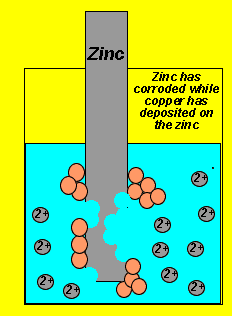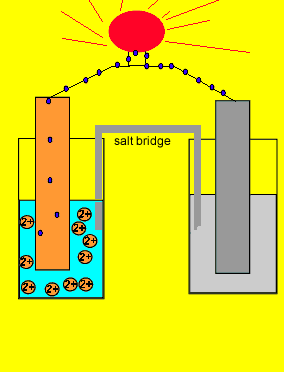Electrical energy from chemical reactions A redox reaction involves two separate reactions, the oxidation (electron giving) and the reduction (electron taking). The reaction pictured on the left is that which occurs when a piece of zinc metal is placed in a solution of copper ions. We notice copper metal being deposited on the zinc metal while the zinc metal simply dissolves or corrodes. The two reactions that are occurring simultaneously are: Oxidation - Zn(s) => Zn+2(aq) + 2e Reduction - Cu+2(aq) + 2e => Cu(s).. In this overall reaction electrons are being transferred from the zinc metal to the copper ions. No useful energy can be attained from this reaction even though electron movement is taking place.Since electrons flow from the zinc electrode we say it is the negative terminal. While the copper metal, where electrons are being attracted to, is said to be the positive electrode. Now if we separate the two reactions and cause the electrons to go from the zinc to the copper ions via an external circuit, useful electrical energy can be achieved. This is exactly what happens in a battery. The two parts of this simple battery are called half cells. The electrode in the half cell where oxidation occurs (electron giving) Zn(s) => Zn+2(aq) + 2e is known as the anode(-). While the electrode in the half cell where electrons are taken Cu+2(aq) + 2e => Cu(s) is known as the cathode(+). Continue